# ML | sklearn.linear_model.LinearRegression() in Python

This is Ordinary least squares Linear Regression from sklearn.linear_module.
Syntax :
sklearn.linear_model.LinearRegression(fit_intercept=True, normalize=False, copy_X=True, n_jobs=1):

Parameters :

fit_intercept : [boolean, Default is True] Whether to calculate intercept for the model.
normalize : [boolean, Default is False] Normalisation before regression.
copy_X : [boolean, Default is True] If true, make a copy of X else overwritten.
n_jobs : [int, Default is 1] If -1 all CPU’s are used. This will speedup the working for large datasets to process.

In the given dataset, R&D Spend, Administration Cost and Marketing Spend of 50 Companies are given along with the profit earned. The target is to prepare ML model which can predict the profit value of a company if the value of its R&D Spend, Administration Cost and Marketing Spend are given.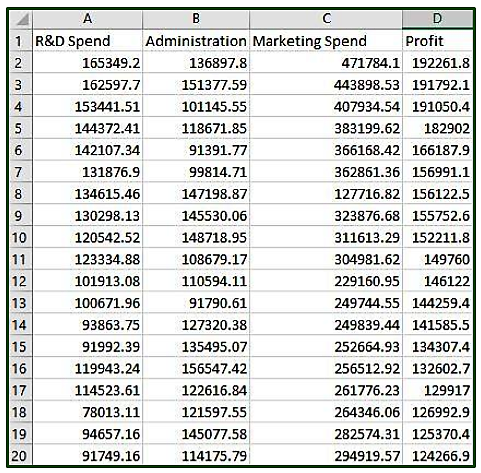Code: Use of Linear Regression to predict the Companies Profit

 `# Importing the libraries ` `import` `numpy as np ` `import` `pandas as pd ` `  `  `# Importing the dataset ` `dataset ``=` `pd.read_csv(``'https://media.geeksforgeeks.org/wp-content/uploads/50_Startups.csv'``) ` `  `  `print` `(``"Dataset.head() \n "``, dataset.head()) ` `  `  `# Input values ` `x ``=` `dataset.iloc[:, :``-``1``].values ` `print``(``"\nFirst 10 Input Values : \n"``, x[``0``:``10``, :]) ` ` `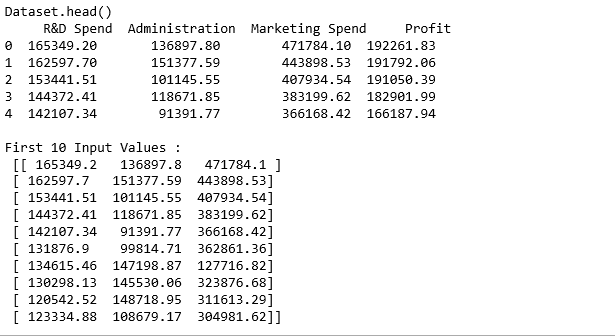`print` `(``"Dataset Info : \n"``) ` `print` `(dataset.info()) `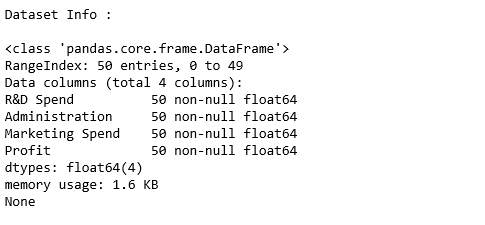`# Input values ` `x ``=` `dataset.iloc[:, :``-``1``].values ` `print``(``"\nFirst 10 Input Values : \n"``, x[``0``:``10``, :]) ` `  `  `  `  `# Output values ` `y ``=` `dataset.iloc[:, ``3``].values  ` `y1 ``=` `y ` `y1 ``=` `y1.reshape(``-``1``, ``1``) ` `print``(``"\n\nFirst 10 Output true value : \n"``, y1[``0``:``10``, :]) `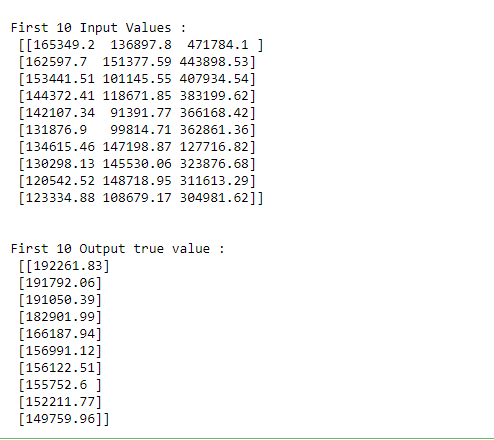`# Dividing input and output data to train and test data ` `# Training : Testing = 80 : 20 ` `from` `sklearn.cross_validation ``import` `train_test_split ` `xtrain, xtest, ytrain, ytest ``=` `train_test_split(x, y, test_size ``=` `0.2``,  ` `                                                     ``random_state ``=` `0``) ` `  `  `# Feature Scaling ` `# Multilinear regression takes care of Feature Scaling ` `# So we need not do it manually  ` `  `  `  `  `# Fitting Multi Linear regression model to training model ` `from` `sklearn.linear_model ``import` `LinearRegression ` `regressor ``=` `LinearRegression() ` `regressor.fit(xtrain, ytrain) ` `  `  `# predicting the test set results ` `y_pred ``=` `regressor.predict(xtest) ` `  `  `y_pred1 ``=` `y_pred ` `y_pred1 ``=` `y_pred1.reshape(``-``1``,``1``) ` `  `  `print``(``"\n   RESULT OF LINEAR REGRESSION PREDICTION : "``) ` `print` `(``"\nFirst 10 Predicted value : \n"``, y_pred1[``0``:``10``, :]) `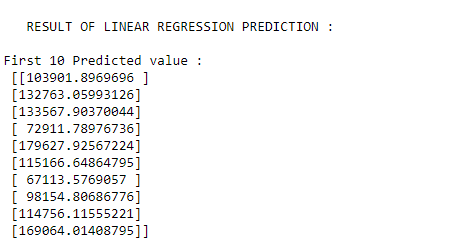My Personal Notes arrow_drop_upIf you like GeeksforGeeks and would like to contribute, you can also write an article using contribute.geeksforgeeks.org or mail your article to contribute@geeksforgeeks.org. See your article appearing on the GeeksforGeeks main page and help other Geeks.

Please Improve this article if you find anything incorrect by clicking on the "Improve Article" button below.